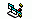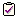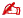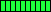Search    VB VB.net C# ASP.net ASP SQL Server VC++ My Links Networking ORACLE MySQL PHP Unix/Linux WinNT/2k/2003 Flash Maya 3D max Photoshop DB2 C/C++/ASM Microcontroller Circuit Design JAVA Wireless/Mobile JScript     Articles from this site only Links only Articles with downloads Articles with screenshot(s)  Code/Page 10 20 30User Login Email Password Remember MeForgot the Password?Services
» Web Development
» Maintenance
» Data Integration/BI
» Information ManagementProgrammingDatabaseAutomationOS/NetworkingGraphicsLinksTools
» Regular Expr Tester
» Free ToolsSimplify and optimize expressions with And, Or and Xor operators

Total Hit ( 2529)Rate this article:     Poor     ExcellentSubmit Your Question/Comment about this article

RatingLet's assume you must test whether the most significan bit of an integer value is set or not. This is the code that you usually write: ' two cases, depending on whether the value is Integer or LongIf intValue And &H8000 Then  ' most significant bit is setEnd IfIf lngValue And &H80000000 Then  ' most significant bit is setEnd IfHowever, all VB variables are signed, therefore the most significant bit is also the sign bit. This means that, regardless of whether you're dealing with an Integer or a Long value, you can test the most significant bit as follows: If anyValue < 0 Then  ' most significant bit is setEnd IfOn the other hand, when you're testing the sign of two or more values you can often simplify and optimize the expression by applying a bit-wise operation to the sign bit. Here are several examples that demonstrate this technique: ' Determine whether X and Y have the same signIf (x < 0 And y < 0) Or (x >= 0 And y >=0) Then ...' the optimized approachIf (x Xor y) >= 0 Then' Determine whether X, Y, and Z are all positiveIf x >= 0 And y >= 0 And z >= 0 Then ...' the optimized approachIf (x Or y Or z) >= 0 Then ...' Determine whether X, Y, and Z are all negativeIf x < 0 And y < 0 And z < 0 Then ...' the optimized approachIf (x And y And z) < 0 Then ...' Determine whether X, Y, and Z are all zeroIf x = 0 And y = 0 And z = 0 Then ...' the optimized approachIf (x Or y Or z) = 0 Then ...' Determine whether any value in X, Y, and Z is non-zeroIf x = 0 And y = 0 And z = 0 Then ...' the optimized approachIf (x Or y Or z) = 0 Then ...It is mandatory that you fully understand how the boolean operators work before using them to simplify a complex expresion. For example, you must be tempted to consider the two following lines as equivalent: If x <> 0 And y <> 0 Then If (x And y) Then ...You can easily prove that they aren't equivalent if using X=3 (binary 0011) and Y=4 (binary 0100). In this case, however, you can partially optmize the expression as follows: If (x <> 0) And y Then ...

 Submitted By : Nayan Patel  (Member Since : 5/26/2004 12:23:06 PM)Job Description : He is the moderator of this site and currently working as an independent consultant. He works with VB.net/ASP.net, SQL Server and other MS technologies. He is MCSD.net, MCDBA and MCSE. In his free time he likes to watch funny movies and doing oil painting. View all (893) submissions by this author  (Birth Date : 7/14/1981 )